Reach Us+44-1522-440391
Effect of Size, Temperature, and Structure on the Vibrational Heat Capacity of Small Neutral Gold Clusters | OMICS International
Journal of Material Sciences & Engineering
All submissions of the EM system will be redirected to Online Manuscript Submission System. Authors are requested to submit articles directly to Online Manuscript Submission System of respective journal.

Effect of Size, Temperature, and Structure on the Vibrational Heat Capacity of Small Neutral Gold Clusters

Vishwanathan K* and Springborg M

Physical and Theoretical Chemistry, University of Saarland, Germany

*Corresponding Author:
Vishwanathan K Physical and Theoretical Chemistry
University of Saarland
66123 Saarbrucken, Germany
Tel: 496813020
E-mail:[email protected]

Received Date: January 24, 2017; Accepted Date: February 27, 2017; Published Date: March 10, 2017

Citation: Vishwanathan K, Springborg M (2017) Effect of Size, Temperature, and Structure on the Vibrational Heat Capacity of Small Neutral Gold Clusters. J Material Sci Eng 6: 325. doi: 10.4172/2169-0022.1000325

Copyright: © 2017 Vishwanathan K, et al. This is an open-access article distributed under the terms of the Creative Commons Attribution License, which permits unrestricted use, distribution, and reproduction in any medium, provided the original author and source are credited.

Visit for more related articles at Journal of Material Sciences & Engineering

Abstract

The vibrational heat capacity Cvib of a re-optimized neutral gold cluster was investigated at temperatures 0.5-300 K. The vibrational frequency of an optimized cluster was revealed by small atomic displacements using a numerical finite-differentiation method. This method was implemented using density-functional tight-binding (DFTB) approach. The desired set of system Eigen frequencies (3N -6) was obtained by diagonalization of the symmetric positive semi definite Hessian matrix. Our investigation revealed that the Cvib curve is strongly influenced by temperature, size, and structure and bond-order dependency. The effect of the range of interatomic forces is studied; especially the lower frequencies make a significant contribution to the heat capacity at low temperatures. In addition to that, we have exactly predicted the vibrational frequencies (ωi) which occur between 0.55 to 370.72 cm-1, depending on the nanoparticle morphology at T=0 for small neutral gold clusters AuN=3-20. This result has been proved and confirmed by the size effect values. It was found that beside the particle size, geometric shape, defect structure and an increase in asymmetry of nanoparticles effects on heat capacity. Surprisingly, the Boson peaks are typically ascribed to an excess density of vibrational states for the small clusters. Finally, temperature dependencies of the vibrational heat capacities of the re-optimized neutral gold clusters have been studied for the first time.

Keywords

Gold atomic clusters; Density-functional tight-binding (DFTB) approach; Finite-differentiation approximation; Force constants (FCs); Vibrational density of states (VDOS); Vibrational heat capacity (Cvib); Boson Peaks (BP)

Introduction

The study of nanostructured materials exhibiting novel properties is one of the most fascinating fields of current research. Small nanomaterial’s are of particular interest because of intriguing characteristics [1-3]. Nanoparticles with smaller dimensions may exhibit different properties in comparison with bulk material. The nanoparticles possess unique physic-chemical, optical and biological proper-ties which can be manipulated suitably for desired applications . The advances in the field of nanoscience and nanotechnology has brought to fore the Nano sized inorganic and organic particles which are finding extensive applications as amendments in medicine and therapeutics, synthetic textiles and food packaging products . The incorporation of engineered nanoparticles into household, personal care, consumer, and industrial products is increasing the exposure of humans and the ecosystems to these materials through production, transportation, storage, use, and disposal. Due to their small sizes, nano-materials (NMs) can enter into cells and interact with cell organelles and/or macromolecules and may thus disrupt the normal cellular functions [6-9]. Various NMs have been observed to show systemic effects when administered into systemic circulation, either intentionally for biomedical therapy or accidentally during environmental exposures, and may even cross the blood/brain barrier [6,8,10].

Clusters are well suited for a rapidly increasing number of applications and they have been an active eld of research for about a quarter of a century. Instead of reviewing all the literature, we refer the reader to the already existing review article by Baletto and Ferrando and also to the book by Wales [11,12]. Clusters can be viewed as solids at the Nano scale; yet molecular cluster chemistry and solid state chemistry have traditionally been considered as separate topics . Nowadays, gold chemistry plays a very important role in Nano electronics and bio-nanoscience . Particularly, gold clusters are of potential relevance to the Nano electronics industry and hence remain the subject of many experimental as well as theoretical studies. They contain edge atoms that have low coordination  and can adopt binding geometries that lead to a more reactive electronic structure . Gold in the Nano regime, especially gold Nano crystals have shown size-sensitive reactive properties and are considered to be as promising chemical catalysts . As one of the precious metals, gold has good corrosion resistance and extremely high stability and has been widely researched for biomedical applications .

The vibrational properties of clusters and small particles have been studied very intensively [19-26], and are vital for understanding and describing the atomic interactions in the cluster [27-32]. Thermal properties like heat capacity and thermal conductivity as well as many other material properties are strongly influenced by the vibrational density of states (VDOS). For this reason, a better understanding of the rules governing the vibrational properties of nanostructured materials is of high technological and must be given a high priority. The vibrational properties play a major role in structural stability [25,26,33].

The size-dependent properties of metallic clusters are currently of considerable interest, both experimentally  and theoretically [24,35]. Although the size effect on specific heat capacity has recently attracted much attention , many publications only focus on low temperature . Some of the theoretical studies on the thermodynamic properties of clusters are based on molecular-dynamic simulations  from which the caloric curve, the heat capacity of clusters and the phase transitions can be determined. Nano clusters are interesting because their physical, optical and electronic characteristics are strongly size dependent. Often changing the size by only one atom can significantly alter the physical chemical properties of the system . Many new periodic tables can thus be envisioned classifying differently-sized clusters of the same material as new elements. Potential applications are enormous, ranging from devices in nano-electronics and nanooptics  to applications in medicine and materials.

Reyes-Nava et al.  calculated the heat capacity for a few NaN systems using the simple many-body Gupta potential, which approximates the atomic interactions with an analytical description that does not explicitly include electronic degrees of freedom. It was found that solid-liquid phase transitions occurring over a certain temperature range depend critically on the size N of the system. Lee et al.  carried out accurate molecular dynamics simulations. From a literature search , one can see that only a very few theoretical studies attempt to calculate the thermodynamically properties of the clusters directly through de-termination of the partition function Z. Doye and Calvo calculated the partition function for Lennard-Jones clusters with N ≤ 150 (Figure 1) .

Figure 1:AuN (N=3-20): The lowest (ωmin) and the highest (ωmax) vibrational frequency as a function of cluster size (N) at T=0 K.

In this study we combine numerical finite-difference approach and DFTB method. At T=0, the vibrational frequency of a re-optimized neutral gold cluster is obtained for optimized cluster of AuN=3-20 which was calculated by Dong and Springborg . The desired set of system Eigen frequencies (3N-6) is obtained by a diagonalization of the symmetric positive semi definite Hessian matrix. The effect of the range of interatomic forces has been studied. We found that the lower frequencies made an excellent contribution to the heat capacity (to be able to store significant heat energy) even at low temperatures (Figure 2). This novel and reliable methodology is constructed to explain the essence of the physical picture.

Figure 2: Cvib(min) per atom as a function of temperature at 0.1-30K. The lowest frequency (ωmin) range: 0.55-9.83 cm-1 for AuN (N=4, 5, 6, 8, 9, 11, 12, 15, 17, 18, 20) and the range:10.08-34.17 cm-1 for AuN (N=3, 7, 10, 13, 14, 16, 19) clusters.

In our case, we are studying the finite-temperature behavior, which is sensitive to the size of the cluster  and the recent interest in planar gold clusters [46,47]. For example, according to Y. Dong and M. Springborg's  results, the gold clusters with up to N = 6 have a two-dimensional structure, whereas from N=7, the gold clusters form three-dimensional structures. However, in some of the research studies say that for the clusters with N=7-15, the structure can be either 2D or 3D or even both. However, experimental and theoretical studies have found that planar structures are stable up to around 15 atoms [48,49].

In the present work, we shall at first remember our earlier results on Vibrational Heat Capacity of Gold Cluster AuN=14 at Low Temperatures . As an extension of that work, we shall subsequently present new results devoted to the vibrational contributions to the thermodynamic low-temperature properties of the other clusters. Nevertheless, the purpose of the last part is to explore which kind of information can be obtained by studying the heat capacities of the AuN=14 cluster and, in particular, to see whether the heat capacities can be correlated to structural and/or energetic properties of the clusters.

Theoretical and Computational Procedure

The DFTB [51-55] is based on the density functional theory of Hohenberg and Kohn in the formulation of Kohn and Sham. In addition, the Kohn-Sham orbitals ψi(r) of the system of interest are expanded in terms of atom-centered basis functions {фm(r)},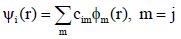(1)

While so far the variational parameters have been the real-space grid representations of the pseudo wave functions, it will now be the set of coefficients cim. Index m describes the atom, where фm is centered and it is angular as well as radially dependent. The фm is determined by self-consistent DFT calculations on isolated atoms using large Slatertype basis sets.

In calculating the orbital energies, we need the Hamilton matrix elements and the overlap matrix elements. The above formula gives the secular equations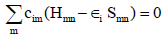(2)

Here, cim's are expansion coe cients,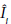is for the single-particle energies (or whereare the Kohn-Sham eigenvalues of the neutral), and the matrix elements of Hamiltonian Hmn and the overlap matrix elements Smn are defined as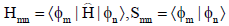(3)

They depend on the atomic positions and on a well-guessed density ρ(r). By solving the Kohn-Sham equations in an effective one particle potential, the Hamil-tonian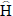is defined as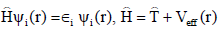(4)

To calculate the Hamiltonian matrix, the effective potential Veff has to be approximated. Here,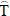being the kinetic-energy operator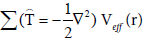being the effective Kohn-Sham potential, which is approximated as a simple superposition of the potentials of the neutral atoms,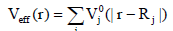(5)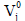is the Kohn-Sham potential of a neutral atom, rj=r-Rj is an atomic position, and Rj being the coordinates of the j-th atom. The short-range interactions can be approximated by simple pair potentials, and the total energy of the compound of interest relative to that of the isolated atoms is then written as,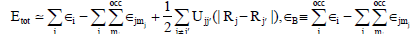(6)

Here, the majority of the binding energy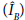is contained in the difference between the single-particle energiesi of the system of interest and the single-particle energies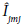of the isolated atoms (atom index j, orbital index mj),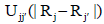is determined as the difference between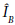and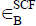for di-atomic molecules (with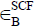being the total energy from parameter-free density-functional calculations). In the present study, only the 5d and 6s electrons of the gold atoms are explicitly included, whereas the rest are treated within a frozen-core approximation [53,55,56].

Re-optimization and numerical force constants (FCs)

The vibrational frequencies of the gold clusters were calculated within the harmonic approximation by diagonalization of the Hessian matrix. The finite-difference method has been implemented within DFTB approach for our calculation (a finite-difference approximation to calculate the force constants). We found a total energy over those gradients were extended for a small displacement ds=(± 0.01) a.u. within the equilibrium coordinates of a previously optimized structure (at T=0) by Dong and Springborg . However, the DFTB method has some difficulties to extract the force constants which are most important for our spectrum calculations. Mainly, to get one set of hessian matrix it is necessary to compute two times for both positive and negative gradients. It is a reasonable value and allowed us to discriminate between the translational, rotational motion (Zeroeigenvalues) and the vibrational motion (Non-Zero-eigenvalues).

In our case, we have calculated the numerical first-order derivatives of the forces (F; F) instead of the numerical-second-order derivatives of the total energy (Etot). In principle, there is no difference, but numerically the approach of using the forces is more accurate,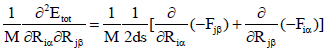(7)

Here, ds is a differentiation step-size and M represents the atomic mass, for homo-nuclear case. The complete list of these force constants (FCs) is called the Hessian H, which is a (3N × 3N) matrix. Here, i is the component of (x, y or z) of the force on the j'th atom, so we get 3N.

Calculation of vibrational heat capacity

Finally, from the calculated vibrational frequencies and with the use of Boltzmann statistics [57-59], we can get the formula to investigate size, structure and temperature effects on the vibrational heat capacity of clusters,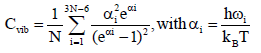(8)

Here, N is the total number of atoms in a cluster, h is the reduced Planck's constant, ωi is vibrational frequencies (low(min); high(max)), kB is Boltzmann's constant and T is the absolute temperature. Naturally, zero frequencies are excluded from summation (3N-6) in eqn. (8). In this study, we focus only on the vibrational part of the heat capacity, i.e., on Cvib.

The Classical Treatment of Ctrans + Crot + Celec: The heat capacity of a system with independent degrees of freedom can be ap-proximated as a sum of its individual contributions,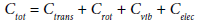(9)

Here, Ctrans, Crot, Cvib and Celec are called as translation, rotational, vibrational and electronic heat capacity. We have proceeded to calculate the specific heat Cvib contribution due to the vibrational energy. In this study, we focus only on the vibrational part of the heat capacity, i.e., on Cvib. The other contributions are treated classically. Most often, the electronic excitation energies are much larger than kT and we neglected the electronic partition function.

Results and Discussion

In this article, we present an in-depth study on the behavior of vibrational frequency at T=0 K, the heat capacity (Cvib) of a reoptimized neutral gold cluster (AuN, N=3-20) at T=0.5 to 300 K and the Boson peaks Cvib/T3 at T=0.5-30 K. Our results are of special interest since the Boson peaks are thermally activated as well as the local atomic arrangements (within a cluster or group of strongly correlated atoms) but still are constrained by surrounding atoms . This is an interesting point and may shed light on the enhancement of the heat conductance process mentioned in Physical Review Letters 103, 048301 (2009)  and PLOS ONE 8, e58770 (2013) . As a function of temperature: at low temperatures the Boson peak corresponds to these local rearrangements with (mutual) reaction of one atom to another .

We applied the method outlined above for studying the properties of neutral gold atomic clusters with sizes AuN=3-20 . We analyzed vibrational spectrum and vibrational heat capacity which is being a striking feature and a classical size effect. Our investigation revealed that the Cvib curve shows a very strong influence of temperature, size and structure dependency. In addition to that we have also plotted the Cvib(min) and Cvib(max) curves separately for the lowest (ωmin) and the highest (ωmax) vibrational frequency modes and then studied their physical behaviors.

We have compared our theoretical results with the theoretical and experimental results calculated by Bishea and Morse , Gruene et al.,  Mancera , Molina et al. , and with Nose , for the vibrational spectrum of AuN=3,4,5,6,7,19,20 clusters. Interestingly, our results were in excellent agreement with their results.

The vibrational frequency (ωi) and properties

Figure 1 shows the low (at the least) and the high (at the most) frequency ranges for cluster AuN=3-20. The lowest and the highest frequency ranges are 0.55-34.18 cm-1 and 165.46-370.72 cm-1, respectively.

The relative importance of high and low frequencies naturally depends on the size, structure and frequency spectra of the clusters. The size is super critical to the physiochemical properties of Au cluster. It is certainly affected by the ratios of dangling bond to overall bulk bonding number. Nanoparticles have a substantial fraction of their atoms on the surface. The surface energy is the (thermodynamically unfavorable) energy of making dangling bonds' at the surface. Atoms at the surface are under-coordinated, and because breaking bonds results in a loss of energy, surface atoms always have higher energy than atoms in the bulk. It is surely expected that such a vibrational spectrum depends on the material, size, and shape of clusters and nanoparticles . Most importantly, the vibrational properties of atomic clusters are a fingerprint of their structures and can be used to investigate their thermodynamic behavior at low temperatures .

Of course, there is an increasing amount literature illustrating the conceptual and practical relevance of two dimensional (2D) systems with long range interactions [69-71]. The vibrational frequency of FCs contributions comes from both the symmetric and the asymmetric stretch and bending modes. We believe that bond-stretching (ks) and bond-bending (kb) force constants depend on the nearest neighbor distance obtained from lattice vibrations . It is important to note that the stretching modes moves to lower frequency (approaching zero), and the bending mode b moves to higher frequency. The low frequencies are probably due to the influence of large collective motions of atoms and the high frequencies due to localized motions of atoms , which diminish bond length fluctuations. The interatomic interaction that is responsible for the frequency ranges and the variation of the FCs led to a shift in the mode frequencies .

The vibrational heat capacity Cvib(min) for the lowest frequency ωmin at 0.1-30 K

Figure 2 has shown Cvib(min) for all the clusters. The frequency of the lowest vibrational state of a nanoparticle is ωmin. The asymptotic behavior of heat capacity at low temperatures takes the form with respect to αiminmin=T of eqn. (8). The ωmin value is determined by the size, shape, and defect structure of the nanoparticle.

Interestingly, for all the clusters the Cvib(min) starting at ranges (critical temperature, Tc) are within the temperature range of about T =Tc=0.1-3 K. As a result, the effect of the lowest frequency modes is dominant for all the clusters. This is very clear evidence that shape of Cvib(min) curves differ with respect to the size and structure of the clusters.

The clusters AuN (N=4, 5, 6, 8, 9, 11, 12, 15, 17, 18, 20) have extremely low ωmin frequencies between 0.55-9.83 cm-1. Which is even below the range of Far Infrared FIR, IR-C 200-10 cm-1? The starting at ranges are within the temperature range of about T=Tc=0.1-0.7 K. In the same manner, for the clusters AuN (N=3, 7, 10, 13, 14, 16, 19) the frequencies are in between 10.08-34.17 cmare within the temperature range of about T=Tc=0.7-3.0 K.. Which is within the range of Far Infrared FIR, IR-C 200-10 cm-1. Due to this the starting at ranges are within the temperature range of about T=Tc=0.7-3.0 K.

In Figure 2, particularly the cluster Au14 (black line) and Au16 (blue line) both are very similar due to their ωmin values, 17.02 and 17.13 cm-1. However, even though they have different sizes, they have the very same Cs symmetry.

Moreover, the Cvib(Min) curve shows the asymptotic behavior, rising smoothly and finally reaching a linear flat at temperatures above T=15 K for all AuN (N=3-20) clusters. This is scientifically significant with respect to the cluster size and shape. The minimum vibrational frequency ωmin plays an important role in determining the shape of the Cvib curve at low temperature.

Anomalous behavior of neutral gold clusters: In Figure 2, we have noticed some anomalous behavior in the shoulder of the heat capacity Cvib(min) curves between the clusters AuN (N=10-19). And the same behavior is exhibited between the clusters AuN (N=4-5), which is due to the size and structure de-pendency of the clusters. The data reveal an anomalous contribution to the heat capacity at low temperatures. It was identified that this anomaly in heat capacity is caused by the effect of disorder in the cluster size and structure. Probably, gold nanostructures exhibit anomalous thermal behavior such as the shape transformation of Nano rods, generation of nanoparticles by heating a mesh etc., at extremely low temperature . The results of our work within the numerical model caused us to consider the intrinsic relation between normal (anomalous) particle diffusion and normal (anomalous) heat conduction, along with size dependent thermal conductivity.

The vibrational heat capacity Cvib(max) for the highest frequency ωmax at 10-1000 K

Figure 3 has shown Cvib(max) for all the clusters. The frequency of the highest vibrational state of a nanoparticle is ωmax. The asymptotic behavior of heat capacity at low temperatures takes the form with respect to αi=ξmax=ωmax/T of eqn. (8). The ωmax value is determined by the size, shape, and defect structure of the nanoparticle.

Figure 3: AuN (N=3-20) cluster: Cvib(max) per atom (for the highest frequency ωmax in cm-1) as a function of temperature at 10-1000 K.

Here at Cvib(max) curve, we did not see much of a difference on the shoulder (asymptotic curve) that means they are very much closer to each other. Of course, here we do not see anomalous behavior as we have observed in the case of low frequency (Figure 2). The reasons are, the high frequencies do contribute to the heat capacity when the temperature increases from 100 to 300 K (Cvib(max)increases from 0.04 kB/ atom to 0.11 kB/atom). Most importantly, as this takes place, vibrational modes with lower frequencies give larger contribution to the heat capacity at ambient temperatures. However, the high frequencies do not generate large Cvib(max) differences when comparing different cluster sizes (Figures 2 and 3). The increase in heat capacity depends on the particle size, shape and the clusters perfection.

In Figure 3 you can see Cvib(max) for all the clusters, and, most importantly, you can see that Cvib(max) starting at ranges are within the temperature range of about T=10-37 K, where the effect of the highest frequency modes are dominant for all the clusters. It is evident, that, the starting points varies with respect to the critical temperature Tc from which the Cvib(max) curve raises either suddenly or smoothly. The overall temperature ranges are 10-1000 K. The maximum high frequency falls within the range of Mid Infrared MIR, IR-C 3330-200 cm-1 for the corresponding harmonic frequency ranges of 165.46-370.72 cm-1, with the one exception of the frequency 165.46 cm-1 which is for the AuN (N=4) cluster.

Comparison: The intensity of the enhancement of the specific heat capacity for the lowest vibrational frequency comes in the temperature range of 0.1-15 K (Figure 2) but for the highest vibrational frequency it covers the temperature span 10-500 K (Figure 3). In both of these cases, above these maximum temperatures it gets saturated. Nevertheless, the vibrational heat capacity Cvib(min) and Cvib(max) is zero at T=0 but there are variations in the at ranges which is only because of the rst mode of the vibrational frequencies, and it nally asymptotes towards 0.125 kB/atom at high temperatures.

Superposition of the lowest, the middle and the highest frequencies

The ESI ϯ shows the frequency ranges for all the clusters, AuN (N=3-20). Figures 4-7 shows how Cvib varies with cluster size, structure and temperature. These results show that Cvib(min) curve for the lowest frequency, by that we mean very low frequency shoulder, varies for different clusters with respect to their size and structure. It is almost identical with the middle Cvib(mid) and the high frequency Cvib(max) curve. These results will be important to describe heat transfer at Nano scale as well as Casimi-Lifshitz forces between clusters due to thermal quantum fluctuations .

Figure 4: Au5,10 cluster: Cvib per atom as a function of temperature at 0.5-300 K. It is a superposition and the contributions from the ωmin and ωmax vibrational modes.

Figure 5: Only for the exact middle frequency (ωmid) as a function of cluster size (N), Au3,5,7,9,11,13,15,17,19 [odd number of clusters] at T=0 K, and the respective modes are 2,5,8,11,14,17,20,23,26. The lowest middle frequency (ωmin) 79.22 cm -1 of Au (N = 19), and the highest middle frequency (ωmax) 106.38 cm -1 of Au (N=7).

Figure 6: Au7,19 cluster (odd): Cvib per atom as a function of temperature at 0.5-300 K. It is a superposition and the contributions from the ωmin, ωmid (exact middle, highest Au7 and lowest Au19 frequency among the 9 odd clusters) and ωmax vibrational modes.

Figure 7: Au4;20 cluster: Cvib per atom as a function of temperature at 0.5-300 K. It is a superposition and the contributions from the ωmin and ωmax vibrational modes.

The lowest frequency: Figure 4 shows the very beginning of the low and the high frequencies, Au (N=5), 0.55 cm-1 and Au (N=10), 34.18 cm-1. However, both the frequency values are only for the lowest frequency range. Here, one of the frequency ranges is not within the Far Region of IR (Far Infrared FIR, IR-C), 200-10 cm-1.

A very interesting observation that Au (N=5) has very high heat capacities (Cvib(min)= ~ 0.125 kB/atom at 5 K), whereas Au (N=10) has low heat capacities (Cvib(min) ≤ 0.01 kB/atom at 5 K). Comparisons of the relative low-frequency modes which were produced at close and distant locations for departures and arrivals at Cvib(min). The effects of temperature on the low-frequency vibrational spectrum and local structural arrangements, and the low-frequency density of states distributions reveal that increasingly transverse atoms motions play a dominant role in controlling the band corresponding to the bending or transverse oscillations of the nano-particles at low temperatures.

The exact middle frequency (only possible for the odd sized clusters): Figure 5 shows that the exact middle frequencies for odd number of clusters, Au3,5,7,9,11,13,15,17,19, out of which Au (N=19), has the lowest middle frequency,79.22 cm-1 and Au (N=7), has the highest middle frequency, 106.38 cm-1 which is the frequency range and the rest of the odd number of clusters which falls within this range (Figures 5 and 6). Here, the frequency ranges are within the Far Region of IR (Far Infrared FIR, IR-C), 200-10 cm-1.

The highest frequency: In the same manner, Figure 7 shows mainly only the very end of low and high frequencies, Au (N=4), 165.46 cm-1 and Au (N=20), 370.72 cm-1. However, both the frequency values are only for the highest frequency range. Here, the frequency ranges are within the Far Region of IR (Far Infrared FIR, IR-C), 200-10 cm-1 and also within the Middle Region of IR (Mid Infrared MIR, IR-C), 3330- 200 cm-1. From the Figures 4-7, we certainly confirmed that the low frequency contributions are much higher than the middle and the high frequency contributions to the heat capacity, Cvib. Amazingly, Cvib curves differ significantly with respect to the low/middle/high frequency as a result of temperature as well as size and structure of the clusters.

The vibrational spectrum (ωi) of AuN=3 and the reliability of our model

Bishea and Morse  did research on the spectrum of Au3. For example, they found that the totally symmetric breathing mode in the excited electronic state had a frequency of 182.9 cm-1. We should expect the totally symmetric breathing mode in the ground state will have a somewhat higher frequency, perhaps around 200-250 cm-1. The gold trimer, Au3, has three normal modes, two of which may be degenerate, depending on the symmetry. Moreover, additional modes may be in other experiments. In our case, after the re-optimization the vibrational frequencies were found to be 19.21, 87.47 and, 246.21 cm-1.

Throughout our calculated vibrational frequencies, we assume that the ground state of Au3 must have either C2v or Cs geometry. We consider the various possible ways that we can arrange the three atoms in Au3, as the Jahn-Teller distortion will drive it away from the equilateral D3h configuration [77-79]. For example, Au3 without the spin-orbit coupling (SOC) effects exhibits a Jahn-Teller distortion towards the C2v symmetry; however, with spin-orbit coupling it recovers the D3h symmetry.

An important point: The very low frequency mode 19.21 cm-1 (Figure 1), corresponds to a motion that is not well-approximated as a harmonic motion, since motion on the potential energy surface along this coordinate converts the molecule from one equivalent isosceles triangle to another. As we move along this coordinate, the potential energy goes up (initially quadratically), then becomes anharmonic, then reaches a maximum, then descends into a different minimum where a different gold atom lies at the apex of the isosceles triangle. This will have implications for the heat capacity (Cvib) (Figure 8), which we have modeled as a pure harmonic oscillator.

Figure 8: Cluster Au3: The Cvib per atom as a function of temperature at 0.5- 300 K. αii/T [violet line] and αii/(0.6950356* T ) of eqn. (8) [green line (Aquamarine 4)] and the corresponding Boson peak Cvib/T3 vs. T.

With this confirmation of Au3 vibrational modes, and with the help of Gabedit package . Tolerance for principal axis classi cation: 0.00500 in angstrom (A) and Precision for atom position: 0.09399 in angstrom (A) we went back to the total energies, structures and then verified their symmetry of global structure optimization which were predicted by Dong and Springborg . As a result, some of those Aun clusters symmetries were differed (For example, Au3 (D2), Au9 (D2v/D2), Au10 (D2), Au11 (Cl), Au12 (Cl ), Au13 (Cs), Au15 (Ci ), Au17 (Cl ), Au18 (C2), Au19 (Cl), and Au20 (Cl)) (Figures 1-6 and 9-14).

Figure 9: Corrected and predicted minima of the global structure optimization of Au3 (C2v) and Au9 (Cs) (from top to bottom) at T=0 K.

Figure 10: Corrected and predicted minima of the global structure optimization of Au10 (S8) and Au11 (C1) (from top to bottom) at T=0 K.

Figure 11: Corrected and predicted minima of the global structure optimization of Au12 (C1) and Au13 (C1) (from top to bottom) at T=0 K.

Figure 12: Corrected and predicted minima of the global structure optimization of Au15 (C1) and Au17 (C1) (from top to bottom) T=0 K.

Figure 13: Corrected and predicted minima of the global structure optimization of Au18 (D1) and Au19 (C1) (from top to bottom) at T=0 K.

Figure 14: Corrected and predicted minima of the global structure optimization of Au20 (C1) at T=0 K.

The discrepancy at the frequency modes: For the global minimum energy structure, if the θ >900 then the modes are 24.7, 127.0, 183.1 cm-1 and if the θ >600 with acute structure the modes are 69.0, 89.3, 167.1 cm-1 again, and if the <600 with acute structure the modes are 5.3, 109.2, 168.6 cm-1, which are Harmonic frequencies for various isomers of Au3 calculated using PBE/VDB by Mancera and Benoit . So this gives a confirmation that the vibrational modes varies with respect to the bond angle and isomers. Even for the experimental values  the modes are 61.9, no value (silent) and 179.9 cm-1 as well as, in another experimental calculation , no value (silent), 118 and 172 cm-1.

The vibrational heat capacity Cvib of gold neutral clusters

Figures 15-17 shows Cvib as a function of temperature for di erent cluster sizes. Out of AuN=3-20 cluster we have selected some of the 14 special clusters which display different interesting properties. The vibrational heat capacity Cvib has been plotted with respect to αii/T and αii /(0:6950356* T ) of eqn. (8) at temperature, T=0.5-300 K.

Figure 15: Au6;7;8: Cvib per atom as a function of temperature at 0.5-300 K.α ii/(0.6950356* T ) of eqn. (8) and the corresponding Boson peak Cvib=T3 vs. T.

Figure 16: AuN (N=3, 4, 10, 13, 14, 15, 18, 20): Cvib per atom as a function of temperature at 0.5-300 K. αii/(0:6950356*T ) of eqn. (8) and the corresponding Boson peak Cvib=T3 vs. T.

Figure 17: Au5, 6, 9, 12: Cvib per atom as a function of temperature at 0.5-300 K. αii/(0.6950356*T ) of eqn. (8). and the corresponding Boson peak Cvib=T3 vs. T.

The Cvib curve is a sum of Cvib curves, one for each normal mode of vibration. Each of these individual Cvib curves is S-shaped, but with the inflection points (where the curve changes from concave up to concave down) occurring at different temperatures. The temperature of the inflection point is proportional to the vibrational frequency for that particular normal mode. Since each individual Cvib curve is S-shaped, the sum must be S-shaped (to a degree) also. However, because our molecules/clusters have some normal modes of vibration with extremely low vibrational frequencies, some of the individual curves being summed to give the total Cvib curve will have their inflection points at extremely low temperatures. If we examined the Cvib curve at extremely low temperatures (perhaps lower than we have calculated, at 0.4 K → 0 K), we would see that they start out at with Cvib=0, then rise from Cvib=0 (concave up), then go through an inflection point at a low temperature. All of this follows logically from the fact that the overall Cvib curve for a single molecule/cluster is a sum of Cvib curves for each vibrational mode.

Au3 (a planar-triangular C2v): In fact, under careful observation, in the Cvib curve, there is a minute \Jerk/bending", in between 0.15 and 0.25 kB/atom at the temperature range about 0.5 to 10 K (Figure 8). Thus, there seems to be a strong correlation between the atom located coordination numbers and the bond angle, which result in a huge variation, due to the fact that the normal mode energy intervals (19.21; 87.47 and; 246.21 cm-1) are so large which has a great influence on the stability of the cluster.

Au6 (a planar-triangular D3h), Au7 (a decahedron D5h) and Au8 (a tetrahedron Td):

In Figure 15, the Cvib curve starts with the temperature at 0.6 K, and the motion of the atom at 10 K, and makes a hump-like shape which is an a fascinating phenomenon to observe. Most importantly, N=6 is a much more rapidly increasing function of T at low temperature than in the cases of the other cluster sizes (but still Cvib rises smoothly and reaches the size effect value). However, it has more energy stored at low temperature, but the Cvib curve crosses over at about 40 K of the Au (N=8) and 50 K of the Au (N=7) clusters which demonstrates its rapidness. Nevertheless, the main reason could be, the minimum frequency start with degenerate, followed by a double state degenerate, followed again double state degenerate and some single state degenerates and finally end with double state degeneracy, which also provides another confirmation that atoms are located in a periodic and zigzag arrangements with respect to the nearest neighboring atoms coordination. In the case of Au7 and Au8 both Cvib starts at 0.5 K and rises smoothly with asymptotic behavior, and these are the only clusters which have more double state degeneracy and triplet state degeneracy, respectively. So the structural dependency of heat capacity is being confirmed with the triple, double and single state degeneracies.

Interatomic interactions in the clusters reflect a frequency distribution with a high degree of degeneracy due to the high symmetry of their lowest energy configurations (the potential energy with respect to the atomic coordinates). No degeneracy was obtained for the other gold clusters (except AuN , (N=6; 7; 8)) due to the absence of symmetry in their minimum energy.

Au4 (D2h); Au10 (S8); Au13 (C1); Au15 (C1); and Au20 (C1): In Figure 16, it is shown that the Cvib curve heat capacity goes sharply up from zero, without the small region where the heat capacity is very close to zero. The very low frequency modes begin contributing to the heat capacity at lower temperatures. It is possible to have very low lying excited electronic states that can be thermally excited at low temperatures. If that is the case, there will be an additional contribution to the heat capacity due to electronic excitation. The heat capacity of the real substance would be higher than what we have calculated. However, no experimental data is available for comparison. Surprisingly, for each size Cvib there is a monotonously increasing function of T (which tends asymptotically). The temperature dependence of the individual modes (Figures 4-7) led to the total vibrational heat capacity for all the clusters.

The calculated heat capacity curve remains near zero at the lowest temperatures, then begins monotonically rising near 1.75 K. Similar behavior is reflected in all of the clusters that were studied, although the precise location of the transition from near-zero values to the monotonic increase varies. The existence of this low-temperature, near-zero heat capacity regions arises because all of these clusters have a lowest vibrational frequency. The heat capacity only begins to deviate significantly from zero when the thermal energy, kT, has a significant likelihood of exciting the lowest frequency vibrational mode. This behavior is in effect a quantum size effect, resulting from the sparse vibrational density of states.

In addition at low temperatures it is the lowest-energy (lowestfrequency) vibrational states that mainly contribute to heat capacity. Indeed, if the frequency of the lowest vibrational state of a nanoparticle is at a minimum, the asymptotic behavior of heat capacity at low temperatures arises. This is a region T=0.5-1.75 K where the effect of the lowest frequency internal modes are dominant for all the clusters.

Au14(Cs): In our case, AuN=14 has Cs symmetric structure and indeed of low symmetry . The vibrational spectrum in structures spans the range from 17.02 up to 240.20 cm-1. The Au14 spectrum includes several low-frequency modes. However, the frequencies are not necessarily all distinct due to degenerations state; i.e., some of the roots of the secular equation may occur more than once. In Figure 16, we show the heat capacity Cvib as a function of T, for N=14 cluster size. As shown by this figure, at very low temperatures (T→1.75-0.5 K) the Cvib curve falls off to zero. Surprisingly, at lower temperatures (1.75-0.5 K) the value of Cvib is very small and close to zero. This shows that some of the modes of vibration of the cluster are frequencies which cannot be accessed at low temperatures.

Figure 16 also shows that interatomic vibrations in heat capacity can be neglected at around (1.75-0.5 K) and the Cvib curve rises gradually with temperature, which is an exact signature of the vibrational changes. The smooth change, especially pronounced at low temperatures (1.75- 25 K), is even more interesting.

It is due to the energy increase of the system. For a given size, the reduction of the heat capacity is more significant at lower temperatures. As the temperature is raised, the difference between the vibrational energies becomes progressively more conspicuous. There can never be any method which explains the difference as long as we retain a simple harmonic oscillator. However, the Cvib curve gives a confirmation that is temperature dependent. In the quantum limit, T (1.75- 0.5 K) →0, the Cvib curve approaches zero, while in the classical limit, T (300 K) →high temperature →size effect, the curve moves towards a value of Cvib=2.50 kB/atom, which clearly indicates a temperature influenced size effect/ dependency.

Au14 and Au14: For Au clusters, Koskinen et al.,  calculated above the ground states of N=11, 12, 13, and 14, respectively, corresponding roughly to T=1000 K. Particularly, they have shown Au-14 as an example to demonstrate the features of a novel liquid-liquid coexistence (LLC), for Au-14 the LLC ends at Etot∼2 eV followed by a 3D-liquid-3D-solid coexistence for Etot<1.1 eV corresponding roughly to temperatures around 300 K (Figure 3c) for the partial caloric curves T (Etot) of both dimensionalities ). The planar clusters form the hot, low-potential energy phase. The 3D liquid phase has the heat capacity of Cvib=4.05 kB and the 3D solid phase Cvib=3.06 kB per atom, which are greater than our estimated value Cvib=2.50 kB=atom at 300 K*, for neutral cluster Au-14.

Moreover, the expected absolute value Cvib should be 2.57 kB/atom. In our case, the difference is only 0.07 kB/atom which are reasonable within the numerical accuracy. Nevertheless, Cvib has been achieved almost an accurate value, 2.56(389) kB/atom when the temperature is high enough, 950 K*. But in the Koskinen et al.,  case, the di erences were higher 1.48 and 0.49 kB/atom than the expected absolute value for both 3D liquid and 3D solid phases. This discrepancy is due to the minimum energy difference of the anionic  and neutral clusters (which are more stable) . So we conclude that the 2D planner structure is preferential over the 3D Structure.

Au18 (D ): In our case, AuN=18 has D symmetric structure and indeed of low symmetry . The vibrational spectra of eigenvalues were found in the region between 8.07 and 232.46 cm-1. In a recent study, Bulusu et al.,  found that hollow gold cages exist for Au with N=16-18 both according to experimental and theoretical results. In the study of Dong and Springborg , they found many AuN clusters with N=7-20 to have cage-like structures. We found that the Cvib curve of a neutral gold cluster increases smoothly towards the high temperatures and approaches a constant value of about Cvib=2.59, in kB/atom at 300 K*, essentially becoming at.

Moreover, the expected absolute value (for size-dependent) should be Cvib=2.67 kB/atom for neutral cluster Au18. In our case, the difference is 0.08 kB/atom from the expected value. Again, nevertheless, Cvib has been achieved almost an accurate value, 2.65(895) kB/atom when the temperature is high enough, 950 K*. With this we can be sure that the structure can be D1 for Au18. However, the Cvib curve shows that the heat capacity goes sharply up from zero, excluding the small region where the heat capacity is very close to zero, at T=0.5-1.25 K. The rest of the clusters size effect has been addressed well.

The acoustic vibrations are more important at low temperatures, because they dominate the heat capacity [20-26]. Low-frequency modes of harmonic systems can be related to the small amplitude of acoustic waves, which are experimentally observed in all elastic bodies. This implies that, at low temperatures, the specific heat is largely determined by the low frequency part of the vibrational spectrum and it is only at high temperatures that a substantial portion of the spectrum comes into play.

The vibrational (Phonon) density of states has been calculated by Sauceda and Garzon . Eigenvectors of normal modes are associated with low and high frequency vibrations. This behavior is caused by the stiffening of the bonds (i.e., the frequency shifts to a higher value as the strength increases). Such a local stiffening of the diagonal terms of the FCs matrix is known to lead to the formation of localized oscillation modes . The nature of the bond is most readily interpreted through the eigenvectors and eigenvalues of this FCs Hessian matrix. The most important contribution is a short-range order in the disordered state of the cluster. FCs (stretching and bending modes) matrices, independent of symmetry and magnitudes, are strongly correlated with the bond lengths. A small change in bond length upon disordering can have a large effect on FCs and vibrational entropy. The general increase in the bond angle in this series indicates an increasing repulsion between the bonds and this is consistent with the increasing bond order.

The contribution of the vibrational free energy is related to the disorder in the FCs. In general, gold clusters are not so strongly disordered, having only minor positional disorder. Whereas the zigzag structure (for nonlinear clusters) has lower energy, in contrast, the zigzag structure within the clusters readily changes into 2D or 3D structures with its nearest neighbors towards better stabilization by multi-coordination . We must remember that each normal mode acts like a simple harmonic oscillator, with a concerted motion of many atoms. The center of mass does not move. All atoms pass through their equilibrium positions simultaneously and normal modes are independent; they do not interact. This means that normal modes do not exchange energy. This is only true in the absence of harmonic terms, which is a theoretical approximation that is never achieved in reality.

For example, if the symmetric stretch is excited, the energy stays in the symmetric stretch. Asymmetry mainly affects the heat capacity at low temperatures; fragmentally both the internal energy and the entropy. Obviously, the Cvib curves are fairly different: the lower frequencies make a larger contribution to heat capacity. The absolute heat capacity value depends strongly not only on the size of cluster, but also on their shape and structural ordering.

Au5(C2v); Au6(D3h); Au9(Cs) and Au12(C1): The energy gap of vibrational modes, the influence of the edge atoms and its effects (\ hump" shape) in Figure 17. The energy intervals are large in between the modes which vary with respect to the cluster size, structure, symmetry, molar mass and the arrangements of bond orders within the clusters. Particularly, at the end of the last two modes of these following clusters. Au5 (C2v): Mode 8 (224.53 cm-1) and Mode 9 (276.83 cm-1), Au6 (D3h): Mode 10 (178.30 cm-1) and degeneracy Modes 11, 12 (282.99 cm-1), Au9 (Cs): Mode 20 (220.93 cm+) and Mode 21 (313.24 cm-1), Au12 (C1): Mode 29 (264.76 cm-1) and Mode 30 (325.89 cm-1). In addition to that the degrees of degeneracy also played a major role, due to the fact of motion of the atoms at the temperature 0.5 to 7 K range, the Cvib curve shows a hump like shape which is an excellent phenomena on these clusters, Au5, Au6, Au9 and Au12 (Figure 17). Thus, we believe that more energy can be stored below 7 K. This also could be the cause of the edge atoms occupying the surface of the clusters.

Thus, in these AuN (N=3-20) cases, frequencies are low and the curve is squeezed so that this point on the curve falls at a low temperature. If the frequencies are much higher, the same point on the curve will fall at a much higher temperature. The heat capacity remains significantly above zero at lower temperatures. This is because the neutral gold cluster has much lower vibrational frequencies than most molecules have. Nevertheless, our investigation revealed, the vibrational heat capacity curve shows a very strong influence of size, temperature, structure and bond-length (stretching, bending) dependency (Figure 18).

Figure 18: The size effect (3N-6)/N of the cluster Au3-20: Cvib per atom (see Table 1 of the ESI ϯ for astric sign*).

The Boson peak (BP) Cvib/T3 vs. T of the neutral gold clusters: As shown in Figures 8, 15-17, with respect to the eqn. (8) and the corresponding Boson peak Cvib/T3 vs. T were plotted at temperature within the range T=0.5-30 K. Truly, we are surprised by our observation of a Boson peak in our nanoparticles. Hao Zhang and Jack F. Douglas [85,86] usually study the Boson peak from the velocity autocorrelation function, but they are both concerned about a \excess" contribution to the vibrational density of states. This feature has been observed in metal nanoparticles and zeolites and attributed to the coordinated harmonic motions of groups of atoms in the boundary region of the particle. There are similarities here to a glass because the surface of a nanoparticle has many features in common with this class of materials. In cases where the modes have been resolved, the Boson peak has corresponded to a ring of oscillating particles, the relatively low mass being related to the relatively high mass of these modes. Farrusseng and Tuel  also studied the perspectives on zeolite-encapsulated metal nanoparticles and their applications in catalysis.

One of the universal features of disordered glasses is the “Boson peak”, which is observed in neutron and Raman scattering experiments. The Boson peak is typically ascribed to an excess density of vibrational states. Shintani and Tanaka  studied the nature of the boson peak, using numerical simulations of several glass-forming systems. They have discovered evidence suggestive of the equality of the Boson peak frequency to the Io e-Regel limit for “transverse” phonons, above which transverse phonons no longer propagate. Their results indicate a possibility that the origin of the Boson peak is transverse vibrational modes associated with defective soft structures in the disordered state. Furthermore, they suggest a possible link between slow structural relaxation and fast Boson peak dynamics in glass-forming systems.

However, Malinovsky and Sokolov  found that the form of a low-frequency Boson peak in Raman scattering is universal for glasses of varying chemical composition. They have shown that from the shape of the Boson peak one can determine the structural correlation function, i.e., the character of violations of ordered arrangement of atoms within several coordination spheres in non-crystalline solids. Most importantly, very recently Milkus and Zaccone found  that bond-orientation order is not so important for the boson peak. Whereas a much more important parameter is the local breaking of inversion symmetry.

Indeed, there is similar behavior of heat capacity in glasses and clusters at low temperatures. Glasses have some distribution of interatomic distances and modification of atomic coordination induced by disorder, compared to periodic crystals . In clusters or nanoparticles, it is caused by the reduced atomic coordination of the surface atoms. Because the ratio of surface to volume is large, the number of atoms with reduced coordination is significant. The vibrations of surface atoms enhance the VDOS at low energies and the heat capacity increases.

The Boson peak is usually studied in glasses, where enhancement of heat capacity is induced by disorder. The modeling of clusters may be important for understanding the mechanism which lead to this effect. In glasses, there are different local con gurations of atoms that may be simulated by isolated clusters. In particular, it was found that the vibrational density of states (VDOS) exhibit an excessively lowfrequency contribution. A corresponding low-temperature peak is observed in the temperature dependence of the specific heat if plotted as Cvib(T )/T3.

Au3(C2v): Figure 8 shows that the increase in Boson peak curve Cvib=T3 from 0-0.00035 kB/atom [violet line] for i=ωi/T and 0-0.001 kB/ atom [green line (Aqua-marine 4)] for αi =i /(0:6950356*T ) found for T→0→high temperature; 30K, and the maximum deviation at 6.00 K and 4.9 K, respectively, are due to a strong disorderly nature in the cluster. In addition to that conformed with the parameter dependency.

Au5(C2v); Au6 (D3h); Au9(Cs) and Au12(C1): Figure 17 shows that the calculated Cvib/T3 vs. T for AuN (N=5; 6; 9; 12) in the temperature 0.5-10 K range. The increase in Boson peak Cvib/T3 from 0.88503 kB/atom (the maximum deviation with higher amplitude at 0.6 K, Au5. Nevertheless, with the lesser amplitude the deviation occurs at 0.6 at 0.6 K for the other clusters, Au6, Au9 and Au12) found for T →0 to higher temperature, the rest of the clusters are in the interval between 0.5 to 4 K.

Howsoever, Figure 16 also shows the calculated Cvib/T3 vs. T for AuN (N = 10; 14; 18; 20) in the temperature 0.5-30 K range. The detailed confirmation is given below

Au10(S8): There is strong evidence that the maximum deviation of the Boson peak Cvib/T3 is 6.88469 × 10-5 kB/atom at 12 K, is lesser amplitude than the all other clusters, which is only because of their large number of high frequency modes.

Au14(Cs): Based on the experimental observations, the maximum in silica glass is placed at about 10 K and 8.5 K for neutral gold cluster (Figure 16 and Richet et al., [92,93]), and the maximum deviation Cvib/ T3 attains 2,4 × 10-4 J/(molK) for silica glass and 0.0004 kB/atom for gold cluster, respectively. Please notice that the units are not the same.

Au18(D1): The Cvib/T3 deviation is 0.00178 kB/atom found for T →3.7 K temperature. It shows the disorder nature of the cluster.

Au20(C1): The Cvib/T3 deviation with a higher amplitude and the intervals in between 5.31 × 10-3-4.97 × 10-3 kB/atom at 1-1.5 K, which has a large number of high frequencies modes.

To be noticed: Let us remember the Figure 2, and the vibrational heat capacity Cvib(min) at above 30 K, that at high temperatures it gets saturated 0.12 kB/atom (as a linear). Similarly, in the Boson peak, Cvib/ T3 also gets saturated (a linear) at above 30 K, but here it is towards zero values (0 kB/atom). The lower frequencies certainly make a larger contribution to heat capacity. Nevertheless, the Boson peaks are highly visible, i.e., and the strength of the peaks strongly depends on the atomic coordination number. There are several origins, most of them related to the thermal fluctuations. If the atomic coordination is low, a single negative force constant renders the atomic arrangement much closer to an unstable situation than in the highly coordinated case .

The size effect of the gold neutral cluster AuN=3-20

Interestingly, from the Figure 18, the clusters Au16 and Au20 possess the most significant and stable cluster of all other clusters which have Cs and C1 symmetry (comparable with Au20 tetragonal cluster). In fact, the cluster Au16 has a greater number of single state degeneracy modes.

Au16: For the size effect at about temperature 950/950* K, the Cvib curve reached 2.62/2.61 kB/atom but for the absolute value it should be 2.63 kB/atom. So there is a minute difference on both Cvib values which is 0.01/0.02 kB/atom.

Au20: For the size effect at about temperature 950/950* K, the Cvib curve reached 2.70/2.69 kB/atom. However, here the absolute value should be 2.70 kB/atom. However, only at T=950*K, there is a minute difference 0.01 kB/atom.

However, this minute differences are also clearly seen at temperature 300/300 K* for the rest of the clusters. The size effect on the heat capacity should be very sensitive to the accuracy because the absolute differences are small as shown in Figure 18.

Physical parameters influence at the Cvib curves and its shapes

If one carefully observes in Figures 2-8 and 15-17 they shows very clearly that the shape of the Cvib curves are not only dependent upon the low and high frequency ranges, but also with respect to the physical parameters (h, kB). However, along with this we have noticed that the size dependency values of Cvib vary a little bit. Nevertheless, with high temperature at 950 K/950 K*, our calculated Cvib values are overlapping with the absolute values of Cvib.

This confirms the accuracy of the size dependency (Figure 18, violet, blue and red line curve). Nevertheless, near to the room temperature at about 300 K/300 K*, the Cvib values are still very close to the absolute values.

Most importantly, at both the above mentioned temperatures, smoothness and the asymptotic behavior of heat capacity Cvib for αii/T, looks like much better than αii / (0:6950356*T) of eqn. (8) (Figures 2, 3 and 8). In addition to that, the changes at the shape of the Cvib curves are only due to existing some single state modes, but many double or triple state degree of degeneracies modes, and they are the contributing factors at Cvib, see for example, cluster sizes Au6,7,8 at T=0 K (Figure 19). So with this in view, we can confidently say that our new methodology is a trustworthy and a successful model, with which one can take one further in making more accurate experimental calculations.

Figure 19: NVM of the Au6,7,8 and Au12,13,14,19,20 and corresponding vibrational frequency (ωi) in cm-1 at T=0 K.

Comparison with the theoretical and experimental results

The spectral frequencies are the most recent studies covering neutral clusters are reported using far-infrared multiphoton-dissociation spectroscopy (FIR-MPD) for AuN=3,4  and for AuN=7,19,20 . In closing we would like to comment on a trend in the experimentally observed low-frequency vibrational spectra which was realized recently by Gruene et al. . This work experimentally investigated neutral gold clusters (AuN=7, AuN=19 and AuN=20) in the gas phase by means of vibrational spectroscopy, which is inherently sensitive to structure. However, before they performed experiments, they used Gaussian package with DFT calculation.

The gold cluster, AuN=7,19,20 has 15, 51, 54 normal vibrational modes (NVM) respectively, some of which may be degenerate, depending on the symmetry. More-over, some modes may appear in one or another experiment. We calculated the normal modes based on the structures which were predicted by Dong and Springborg . However, after re-optimization the vibrational frequencies were found as mentioned in the Figures 2-19. Our calculated spectrum ranges are the peculiar values, which are in excellent agreement with the theoretical (DFT) and experimental results calculated for gold clusters by Gruene et al. . Their visible modes at IR absorption coefficients, and the (ωi) frequencies are Au20 (148 cm-1), Au19 (149 and 166 cm-1), and for Au7 (165, 186 and 201 cm-1). These values are in excellent agreement with our calculated value of Au20 (148.64 cm-1) and for Au19 (within 144.15, 152.87 158.47, 165.28 cm-1) (Figure 19).

However, they have only predicted vibrational frequencies in between 47 and 220 cm-1 wavenumbers. We have calculated even lower (3.99 cm-1) and higher (370.72 cm-1) wavenumbers than those. This is because in infrared (IR) absorption spectroscopy the number of allowed transitions is restricted by selection rules, and thus directly reflects the symmetry of the particle.

Overall for cluster AuN=3-20, certainly and in particularly, our lowest minimum frequency value of Au8 cluster is 4.66 cm-1 closer to the harmonic frequency of the Au8 cluster which was calculated by Mancera and Benoit . Their harmonic frequency is 6.9 cm-1, as a minimum. Additionally, our highest maximum frequency values are comparable with Nose S calculated (ωi=221 cm-1) as a highest frequency for Au20 Tetrahedron symmetry  using Molecular Dynamics Simulations.

Our calculated Au20(C1) cluster's vibrational modes are comparable and in excellent agreement with the Au20 Tetrahedron (Td) symmetry calculated using density functional theory (DFT) with two different approximations: the hybrid-B3LYP and GGA-BP86 by Molina et al. . Their ranges for BLYP and BP86 are 26.42-162.22 cm-1 (BLYP) and 29.13-172.35 cm+ (BP86), respectively. Certainly, the most stable structure for Au20 was found through frequency calculations for the (Td) Au20 structure by Jun Li, Xi Li, Hua-Jin Zhai, and Lai-Sheng Wang [95,96].

The harmonic frequencies (ωi) for the global minimum energy structure of Au4,5 calculated using PBE/VDB, in cm-1 are being reported by Mancera and Benoit , which are in good agreement with some of our calculated normal modes of Au4 (a planar rhombus D2h): 165.46 (167.2) cm-1; 147.12 (152.2) cm-1; 98.00 (96.5) cm-1 and 31.63 (34.5) cm-1, as well as, Au5 (a planar trapezoid C2v): 132.74 (137.5) cm-1; 47.70 (47.6) cm-1 and 43.25 (47.6) cm-1.

Moreover, our verified symmetry and calculated vibrational frequency modes of AuN=3-6 clusters are almost in complete agreement with Mancera and Benoit .

AuN=6-8: Double and Triple state degeneracy at T= 0

These clusters are unique among the other clusters, due to their nature. Very strong and visible evidence of the vibrational heat capacity Cvib per atom as a function of temperature is shown in Figure 15.

AuN=6 (D3h): The harmonic frequencies for the Au6 cluster are in the range of 2.44 cm-1 to 282.99 cm-1, and there are 3 doubled and 1 tripled degenerate frequencies. 12 normal modes are represented as the following, one mode with degeneracy 3, three modes with degeneracy 2, and three single modes with non-degeneracy.

AuN=7 (D5h): The harmonic frequencies for the Au7 cluster the range of 20.48 cm-1 to 235.19 cm-1, and there are only 6 doubly degenerate frequencies, which is an exceptional case of all other clusters. Out of 15 normal modes, 12 of these modes are twofold-degenerate and the remaining 3 are non-degenerate.

AuN=8 (Td): The harmonic frequencies for the Au8 cluster are in the range of 4.66 cm-1 to 215.08 cm-1, there are 2 doubly and 4 triply degenerate frequencies. Out of 18 modes, 4 threefold, 2 double fold and 2 non-degenerate normal frequencies occurred.

Conclusions with Perspective

We have extracted vibrational frequencies at T=0 K, and investigated the vibrational heat capacities at a temperature range 0.5-300 K, of the re-optimized gold atomic clusters (AuN=3-20). Especially, the vibrational modes with lower frequencies are given a significant contribution to the heat capacity at low temperatures. The Cvib is a monotonic function that tends asymptotically. The vibrational heat capacity is a strong function of cluster size and temperature, particularly in the low temperature regime.

Our first step towards the understanding of the so-called finite temperature effects was to take into account the vibrations of clusters. We focused our interest on the cluster size and temperature dependence of the heat capacities and the free energies of the clusters. Finally, we have studied vibrational and thermodynamic properties of the clusters in order to explore the interaction between stability, structure, and heat capacities of clusters. Our approach is worthy of further investigation and would pave a way in realizing numerical values which would allow for an experimental vibrational spectrum and heat capacity, which would prove crucial in development of Nano electronic devices. Nevertheless, our work gives a possible cause for the size, temperature, and structures effect of Au atomic clusters.

Supplementary Material

Details about the harmonic frequencies and the predicted minima of the global structure optimization of Au clusters can be found in the supplementary material (see the ESIϯ).

Acknowledgements

A part of this work was supported by the German Research Council (DFG) through project Sp 439/23-1. We gratefully acknowledge their very generous support.

References

Select your language of interest to view the total content in your interested language

Article Usage

• Total views: 1784
• [From(publication date):
April-2017 - Jun 26, 2019]
• Breakdown by view type
• HTML page views : 1685Can't read the image? click here to refresh